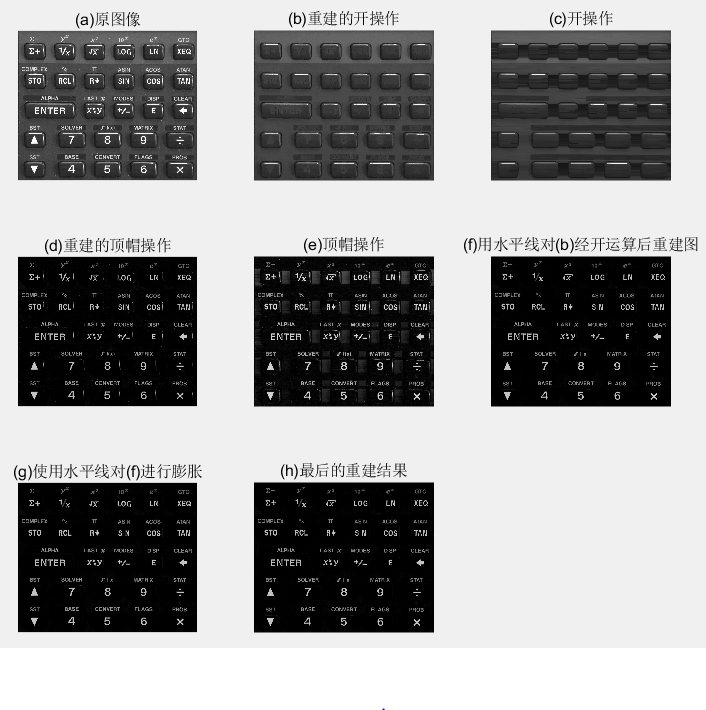2017-05-12 13:34:22 qq826309057 阅读数 772
• ###### MATLAB图像处理

全面系统的学习MATLAB在图像处理中的应用

20258 人正在学习 去看看 魏伟

## 前言

连通域标记是二值图像分析中非常重要的一种方法，也是其他二值图像处理的基础与前提。
所谓连通域标记，就是将一副二值图像中的每个白色像素进行标记，属于同一个连通域的白色像素标记相同，不同连通域的白色像素有不同的标记，从而能将图像中每个连通域提取出来。
连通域标记的算法有很多种，这里介绍其中一种基于一次遍历图像，记录等价对的标记方法，这种方法效率很高。

## 算法原理

### 4 邻接与 8 邻接

在介绍算法前，我们先了解一下什么算是连通。如果两个白色像素邻接，则我们认为这两个像素是连通的；同时，若像素 A 与像素 B 连通，像素 B 与像素 C 连通，则像素 A 与 C 也是连通的。
因此，我们需要定义什么样的两个像素点算是邻接的。常见的邻接关系有两种：4 邻接与 8 邻接。如下图所示：### 遍历图像

for 二值图像中的每一行: //列也可以
1. 记录此行白色像素的每一个序列的的起始位置，终止位置；
2. 除第一行以外(第一行直接标记)，判断是否与上一行序列有重叠：
如果没有重叠，则分配一个新的标记；
如果有一个重叠，则用上一行序列的标记进行标记；
如果有一个以上的重叠，则用上一行重叠序列中最小的进行标记，同时将后面几个标记与此标记记为等价对；
end### 消除等价对

在上一步中，我们得到了若干个等价对，每一个等价对 <a,b> 表示被标记 a 区域与被标记 b 的区域是连通的，因此，我们希望将每个等价对中的标记更新为同一个标记。用图的遍历即可做到这一点。
我们将每一个标记看作一个图的结点，每一个等价对看作是图的边，我们要做的就是通过图的遍历来寻找属于同一个最大连通子图的结点。这些结点锁表示的标记是等价的。## 代码

#include <opencv2\opencv.hpp>
#include <iostream>
#include <utility>
#include <vector>
#include <algorithm>
#include <ctime>
#include <list>
#include <queue>
#define HEIGHBOR_4 4
#define HEIGHBOR_8 8
#define UPWARDFIND 1
#define DOWNWARDFIND 2
using namespace std;
using namespace cv;

struct Group {
int start;
int end;
int tag;
};
//寻找一个group与上一行的group是否有重叠，返回值若为-1，则表示没有重叠，若为其他值，则返回值是这个group的标记，同时记录等价对
int findEqualPair(vector<pair<int,int>> &_equal_pairs, Group _group,const vector<Group> &_pre_groups,int _mode)
{
vector<int> tags;
if (_mode == HEIGHBOR_4)
{
for (auto i : _pre_groups)
{
if (!(i.start > _group.end || _group.start > i.end))
tags.push_back(i.tag);
}
}
if (_mode == HEIGHBOR_8)
{
for (auto i : _pre_groups)
{
if (!(i.start+1 > _group.end && _group.start+1 > i.end))
tags.push_back(i.tag);
}
}
if (tags.size() == 0)
{
return -1;
}
sort(tags.begin(), tags.end());
tags.erase(unique(tags.begin(), tags.end()), tags.end());
int min_tag = tags;
for (int i =1;i<tags.size();i++)
{
_equal_pairs.push_back(pair<int, int>(min_tag, tags[i]));
}
return min_tag;

}
//连通域标记
int tagDomain(Mat &_image,Mat &_result,int _mode)
{
int width = _image.cols;
int height = _image.rows;
_result = Mat(_image.size(),CV_16UC1, Scalar::all(0));
vector<vector<Group>> groups;//用于存团
vector<pair<int, int>> equal_pairs;//存储等价对
int tag_count = 0;
//第一次遍历
clock_t time1, time2;
for (int i = 0; i < height; i++)
{
vector<Group> tmp_groups;
uchar* row = _image.ptr<uchar>(i);
int j = 0;
time1 = clock();
while (j < width)
{
if (row[j] == 255)
{
Group group;
group.start = j;
j++;
while (row[j] == 255 && j < width)
{
j++;
continue;
}
group.end = j - 1;
if (i != 0)
{
int tmp_tag = findEqualPair(equal_pairs, group, groups[i - 1], _mode);
if (tmp_tag != -1)
group.tag = tmp_tag;
else
group.tag = ++tag_count;
}
else
{
group.tag = ++tag_count;
}
tmp_groups.push_back(group);
//cout << "(" << group.first << "," << group.end << "):" << group.tag << endl;
}
else
{
j++;
}
}
time2 = clock();
groups.push_back(tmp_groups);
}
//消除等价对（图的遍历）
//消除重复的等价对
sort(equal_pairs.begin(), equal_pairs.end());
equal_pairs.erase(unique(equal_pairs.begin(), equal_pairs.end()), equal_pairs.end());
//构建图
int size = tag_count+1;
vector<list<int>> graph(size);
vector<int> updata_tag(size,0);
for (auto equal_pair : equal_pairs)
{
graph[equal_pair.first].push_front(equal_pair.second);
graph[equal_pair.second].push_front(equal_pair.first);
}
//遍历
tag_count = 1;
int first_node;
bool finished = false;
while (true)
{
finished = true;
for (int i = 1; i < size; i++)
{
if (updata_tag[i] == 0)
{
finished = false;
first_node = i;
break;
}
}
if (finished)
break;
//图的广度优先遍历
queue<int> q;
updata_tag[first_node] = tag_count;
q.push(first_node);
while (!q.empty())
{
int tmp_node = q.front();
q.pop();
for (auto i : graph[tmp_node])
{
if (updata_tag[i] == 0)
{
updata_tag[i] = tag_count;
q.push(i);
}
}
}
tag_count++;
}
//
for (int i = 0; i < height; i++)
{
ushort* data = _result.ptr<ushort>(i);
for (auto j : groups[i])
{
int tmp_tag = updata_tag[j.tag];
for (int n = j.start; n <= j.end; n++)
{
data[n] = tmp_tag;
}
}
}
return tag_count-1;
}
//随机取色
Scalar random_color(RNG &_rng)
{
int icolor = (unsigned)_rng;
return Scalar(icolor & 0xFF, (icolor >> 8) & 0xFF, (icolor >> 16) & 0xFF);
}
//给连通域涂上不同的颜色
Mat drawDomain(Mat &_domain_tag, int _num, RNG &_rng)
{
Mat result(_domain_tag.size(), CV_8UC3, Scalar::all(0));
vector<Scalar> colors;
for (int i = 0; i < _num; i++)
{
colors.push_back(random_color(_rng));
}
int height = result.rows;
int width = result.cols;
for (int i = 0; i < height; i++)
{
Vec3b* data = result.ptr<Vec3b>(i);
ushort* tag_data = _domain_tag.ptr<ushort>(i);
for (int j = 0; j < width; j++)
{
Scalar color = colors[tag_data[j]-1];
data[j] = color;
data[j] = color;
data[j] = color;
}
}
return result;
}

int main()
{
Mat binary;
cvtColor(image, binary, CV_BGR2GRAY);//图像本身是二值图，转下灰度就可以了
clock_t time1, time2;
time1 = clock();
Mat result;
int num = tagDomain(binary, result, HEIGHBOR_4);
Point seed1, seed2;
seed1 = Point(2000, 2150);
seed2 = Point(2000, 350);
Point seam_point_down = findSeam(binary, seed1, DOWNWARDFIND);
Point seam_point_up = findSeam(binary, seed2, UPWARDFIND);
cout << num << endl;
Mat draw_result = drawDomain(result, num, RNG(123));
imwrite("images\\draw_result.bmp", draw_result);
imshow("draw_result", draw_result);
waitKey(0);
return 0;
}

## 结果2017-05-19 19:46:21 guyuealian 阅读数 17147
• ###### MATLAB图像处理

全面系统的学习MATLAB在图像处理中的应用

20258 人正在学习 去看看 魏伟

# Matlab形态学图像处理：二值图像分割 标记连通区域和重心位置 删除连通区域

【尊重原创，转载请注明出处】http://blog.csdn.net/guyuealian/article/details/71440949
    Matlab中可以使用graythresh(Img)函数设置二值化的阈值，再用im2bw转化为二值图像。在Matlab中，可以使用bwlabel()和bwlabeln()函数来标记二值图像的连通区域。需要注意的是：所谓的连通区域标记是指对二值图像中白色像色而言，即值为1的像素进行标记，而黑色像素看作是背景颜色。当然，Matlab中还有个regionprops()函数可以用于统计图像区域的属性，如面积大小，重心位置。关于bwlabel()、bwlabeln()和regionprops()的用法，请查看相关博客吧

    本博客Matlab代码将实现的功能：将图像转为二值图像，分割出感兴趣的区域，并用“红色矩形线框”标记连通区域的面积，用“蓝色点”标记连通区域的重心位置，为了减少噪声的干扰，代码中将连通区域面积（像素个数）不足100的区域认为是噪声点，并将其删除（即置为背景黑色）。本人用PS制作了一个GIF动画图，以便大家观看效果图：clc;clear all;close all
%% 清空变量，读取图像，并显示其属性
clear;close all
%显示原始图像
figure,
subplot(2,2,1),imshow(src),title('原图')

%用ostu方法获取二值化阈值，进行二值化并进行显示
level=graythresh(src);
bw=im2bw(src,level);
subplot(2,2,2),imshow(bw),title('二值图像')

%运用开操作消去噪点
se = strel('disk',2);
openbw=imopen(bw,se);%对白色点而言
subplot(2,2,3),imshow(openbw),title('开运算后的效果图')

%获取连通区域，并进行显示
% L = bwlabel(openbw,8);
[L,num] = bwlabel(openbw,8);
RGB = label2rgb(L);
subplot(2,2,4),imshow(RGB),title('用rgb颜色标记不同区域')

%获取区域的'basic'属性， 'Area', 'Centroid', and 'BoundingBox'
% stats = regionprops(openbw, 'basic');
stats = regionprops(openbw, 'BoundingBox' ,'Area','Centroid' ,'PixelList' ); %统计白色的连通区域
centroids = cat(1, stats.Centroid);

%%
noiseArea=100;
figure,imshow(openbw),title('2')
hold on
for i=1:size(stats)
imshow(openbw)
rectangle('Position',[stats(i).BoundingBox],'LineWidth',2,'LineStyle','--','EdgeColor','r'),
plot(centroids(i,1), centroids(i,2), 'b*');             %每个连通区域的重心位置
area = stats(i).Area;                                   %连通区域的面积
if area<noiseArea                                       %若当前连通区域面积小于噪声点的面积，则该区域设置为0
pointList = stats(i).PixelList;                     %每个连通区域的像素位置
rIndex=pointList(:,2);cIndex=pointList(:,1);
pointList = stats(i).PixelList;                     %连通区域的像素坐标
openbw(rIndex,cIndex)=0;                            %连通区域的面积不足100，置为背景颜色
end
pause(1);
saveas(gcf,sprintf('img/%d',i),'jpg')                   %保存图片
end
hold off2017-08-25 15:35:37 qq_26035563 阅读数 425
• ###### MATLAB图像处理

全面系统的学习MATLAB在图像处理中的应用

20258 人正在学习 去看看 魏伟

void MergeRuns(vector<pair<int,int>>equivalences,  vector<vector<int>>&overlap)
{
for(int i=0; i<equivalences.size();i++)
{
vector<int>cur;     //存放当前正在合并的团

//初始化当前合并的团（即把第一个未合并的等价对放入其中）
int first = equivalences[i].first;
int second = equivalences[i].second;
cur.push_back(first);
cur.push_back(second);

//从第一个未合并的等价对之后开始对比判断
for(int j=i+1;j<equivalences.size();j++)
{
int pre_size = cur.size();  //当前合并团在该次合并前的大小
for(int k=0;k<cur.size();k++)
{
//判断当前合并团是否与当前等价对有重叠，
//如果重叠，则将另一团标号放入当前合并团，并跳出循环
if(cur[k] == equivalences[j].first)
{
cur.push_back(equivalences[j].second);
vector<pair<int,int>>::iterator iter = equivalences.begin()+j;
equivalences.erase(iter);
iter = equivalences.begin();
break;
}
else if(cur[k]==equivalences[j].second)
{
cur.push_back(equivalences[j].first);
vector<pair<int,int>>::iterator iter = equivalences.begin()+j;
equivalences.erase(iter);
iter = equivalences.begin();
break;
}
}

//判断此次合并是否有新团标号加入，
//如果是，则需从第一个未合并的等价对之后开始对比判断
if(pre_size!=cur.size())
{
j=i;
}
}
overlap.push_back(cur);     //将当前合并完成的团放入结果vector中
}

}1. 产生的结果没有进行排序（想来不会影响最终标记，便未加优化）
2. 再者，在进行合并时未加入单独团标号，即不存在等价对的团标号，比如本例中的4号。

2017-8-29修改

//添加参数int label_num，表示团标号最大值
void MergeRuns(vector<pair<int,int>>equivalences,  vector<vector<int>>&overlap, int label_num)
{
...
//以上为之前代码，未做修改
//下面为添加无等价对的团标号代码
//处理方式其实很无脑，就是对所有团标号进行查找，
//找到不在合并后的团中的就将其放入结果vector中
for(int i = 0;i<=label_num;i++)
{
for(int j=0;j<overlap.size();j++)
{
int k=0;
for(;k<overlap[j].size();k++)
if(overlap[j][k]==i)
break;
if(k<overlap[j].size())
break;
else if(j==overlap.size()-1)
{
vector<int>cur;
cur.push_back(i);
overlap.push_back(cur);
}
}
}
}2017-11-10 20:28:27 hustllx 阅读数 1765
• ###### MATLAB图像处理

全面系统的学习MATLAB在图像处理中的应用

20258 人正在学习 去看看 魏伟

1.使用栈来实现区域标记代码：

close all;clear all;clc;
s=im2bw(I);
L8=bwlabel(s,8);

[m n]=size(s);
tmp=zeros(m,n);

label=0;            %遍历时标记的标签数量
for i=1:m
for j=1:n
cur=s(i,j);
if cur==1&&tmp(i,j)==0             %只有还未遍历过的值为1的才可以进入
label=label+1;
tmp(i,j)=label;
k=1;
stack(k,1)=i;stack(k,2)=j;       %类似于栈的作用，遍历一个区块的，栈的每一个元素为两个，栈的长度未定
while(isempty(stack)==0)      %只有全部遍历完才退出栈，同时这些遍历过的元素不会再遍历
i1=stack(k,1);j1=stack(k,2);
stack(k,:)=[];
k=k-1;                   %k表示在栈中的位置,此处为弹出元素
if i1-1>0&&s(i1-1,j1)==1&&tmp(i1-1,j1)==0 %原图像当前像素上方元素
tmp(i1-1,j1)=label;
k=k+1;
stack(k,1)=i1-1;stack(k,2)=j1;
end
if i1+1<=m&&s(i1+1,j1)==1&&tmp(i1+1,j1)==0%原图像当前像素下方元素
tmp(i1+1,j1)=label;
k=k+1;               %此处为在栈中加入元素
stack(k,1)=i1+1;stack(k,2)=j1;
end
if j1-1>0&&s(i1,j1-1)==1&&tmp(i1,j1-1)==0%原图像当前像素左面元素
tmp(i1,j1-1)=label;
k=k+1;
stack(k,1)=i1;stack(k,2)=j1-1;
end
if j1+1<=n&&s(i1,j1+1)==1&&tmp(i1,j1+1)==0%原图像当前像素右面元素
tmp(i1,j1+1)=label;
k=k+1;
stack(k,1)=i1;stack(k,2)=j1+1;
end
%左上元素
if i1-1>0&&j1-1>0&&s(i1-1,j1-1)==1&&tmp(i1-1,j1-1)==0
tmp(i1-1,j1-1)=label;
k=k+1;
stack(k,1)=i1-1;stack(k,2)=j1-1;
end
%右上元素
if i1-1>0&&j1+1<=n&&s(i1-1,j1+1)==1&&tmp(i1-1,j1+1)==0
tmp(i1-1,j1+1)=label;
k=k+1;
stack(k,1)=i1-1;stack(k,2)=j1+1;
end
%左下元素
if i1+1<=m&&j1-1>0&&s(i1+1,j1-1)==1&&tmp(i1+1,j1-1)==0
tmp(i1+1,j1-1)=label;
k=k+1;
stack(k,1)=i1+1;stack(k,2)=j1-1;
end
%右下元素
if i1+1<=m&&j1+1<=n&&s(i1+1,j1+1)==1&&tmp(i1+1,j1+1)==0
tmp(i1+1,j1+1)=label;
k=k+1;
stack(k,1)=i1+1;stack(k,2)=j1+1;
end

end
end
end
end

2.使用递归的方式来实现：

2019-05-11 08:31:12 Dujing2019 阅读数 1238
• ###### MATLAB图像处理

全面系统的学习MATLAB在图像处理中的应用

20258 人正在学习 去看看 魏伟

# 数字图像处理—形态学图像处理

## （一）预备知识

1. 用数学形态学（也称图像代数）表示以形态为基础对图像进行分析的数学工具
2. 基本思想是用具有一定形态的结构元素去度量和提取图像中的对应形状以达到对图像分析识别的目的
3. 形态学图像处理的数学基础和所用语言是集合论
4. 形态学图像处理的应用可以简化图像数据， 保持它们基本的形状特性，并除去不相干的结 构
5. 形态学图像处理的基本运算有4个：膨胀、 腐蚀、开操作和闭操作

#### 1.1 集合理论中的基本概念

1. 所有像素坐标的集合均不属于集合A，记为$A^c$，由下式给出: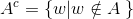这个集合称为集合A的补集

2. 集合B的反射，定义为：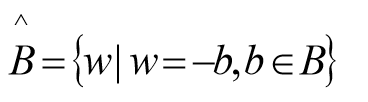即关于原集合原点对称 .

3. 集合A平移到点z=(z1,z2),表示为(A)z，定义为: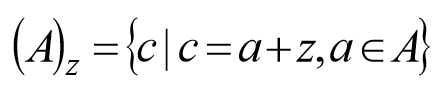#### 1.2 二值图像、集合及逻辑算子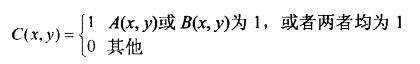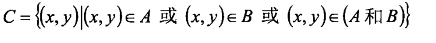1. A为图像集合，B为结构元素（集合）。
2. 数学形态学运算时B对A进行操作。
3. 结构元素要有1个原点（即结构元素参与形态学运算的参考点），可以是中心像素，原则上可选任何像素。
注意：原点可以包含在结构元素中，也可以不包含在结构元素中，但运算的结果常不相同。

f = imread('D:\数字图像处理\第九章学习\Fig0903(a).tif');
subplot(2,3,1), imshow(f);title('(a)二值图像 A:');
subplot(2,3,2), imshow(g);title('(b)二值图像 B:');
subplot(2,3,3), imshow(~f);title('(c)A的补集~A:');
subplot(2,3,4), imshow(f|g);title('(d) A和B的并集 A|B:');
subplot(2,3,5), imshow(f&g);title('(e)A和B的交集 A & B:');
subplot(2,3,6), imshow(f&~g);title('(f)A和B的差集 A&~B');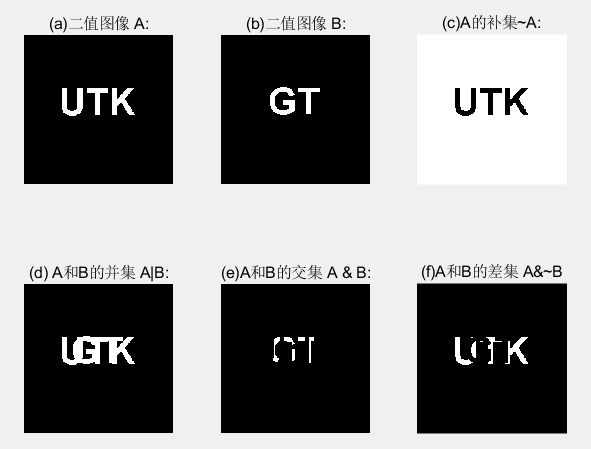## （二）膨胀和腐蚀

#### 2.1 膨胀

A和B是两个集合，A被B膨胀定义为：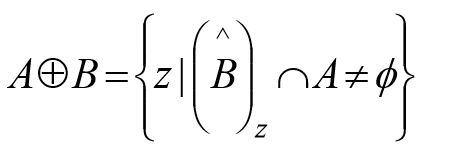1. B的反射进行平移与A的交集不为空。
2. B的反射：相对于自身原点的映象。
3. B的平移：对B的反射进行位移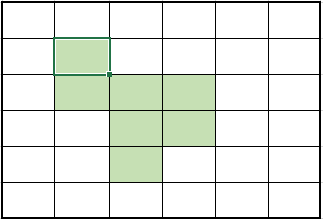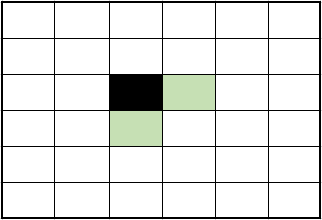(a)集合A    (b)结构元素B (黑色为原点所在)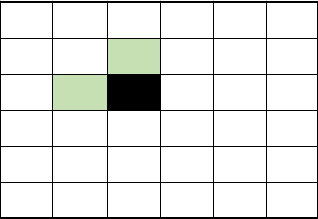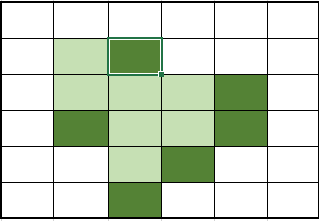(c)结构元素B的映像    (d)图中两种阴影部分（深色为扩大的部分）合起来为A+B

1. 膨胀运算只要求结构元素的原点在目标图像的内部平移，换句话说，当结构元素在目标图像上平移时，允许结构元素中的非原点像素超出目标图像的范围
2. 膨胀运算具有扩大图像和填充图像中比结果元素小的成分的作用，因此在实际应用中可以利用膨胀运算连接相邻物体和填充图像中的小孔和狭窄的缝隙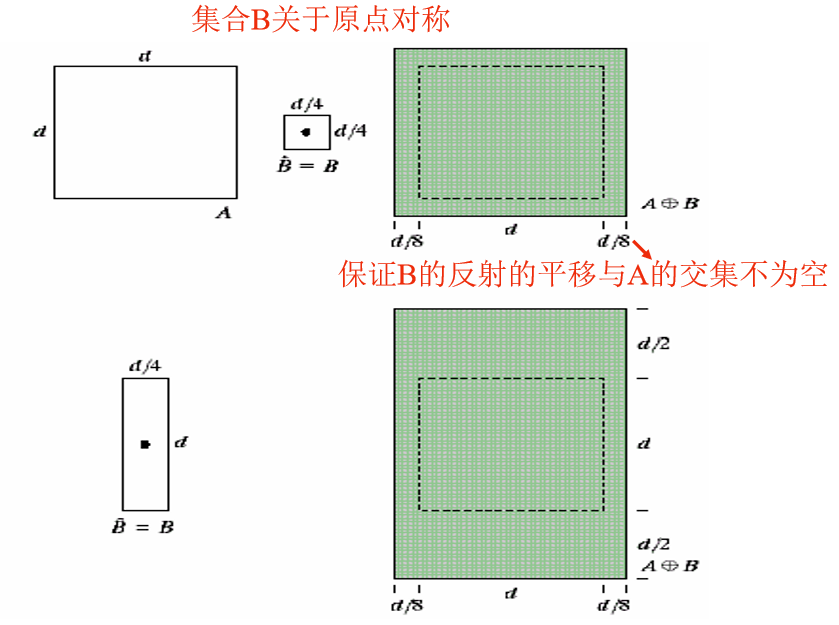D = imdilate(A,B)

A = imread('D:\数字图像处理\第九章学习\Fig0906(a).tif');
B = [0 1 0; 1 1 1; 0 1 0];   %指定结构元素由0和1组成的矩阵
A2 = imdilate(A, B);    %二值图像
subplot(1,2,1), imshow(A);title('(a)包括断开文本的输入图像:');
subplot(1,2,2), imshow(A2);title('(b)膨胀后图像:');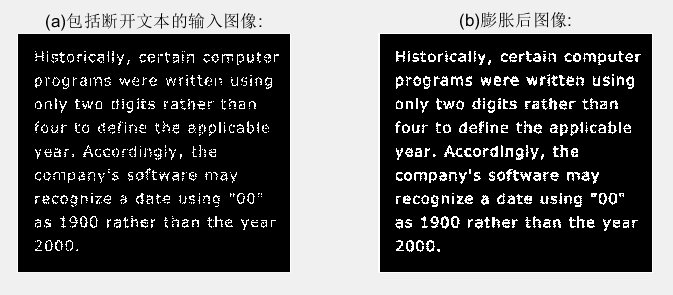#### 2.2 结构元的分解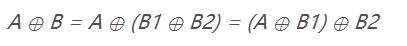B膨胀A等同于B1先膨胀A，再用B2膨胀之前的结果。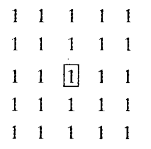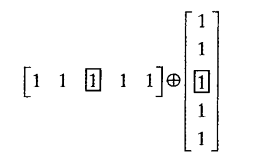#### 2.3 strel函数

se = strel(shape, parameters)

shape用于指定希望形状的字符串，parameters是描述形状信息的参数列表。

#### 2.4 腐蚀

A和B是两个集合，A被B腐蚀定义为：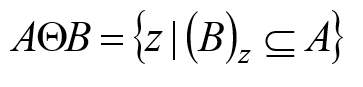1. A被 B 腐蚀是包含在A中的B由z平移的所有点z的集合。
2. B包含在A中的声明相当于B不共享A背景的任何元素。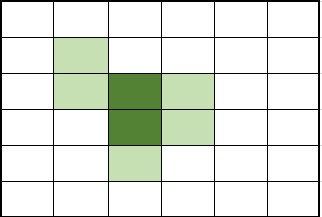(a)集合A(阴影部分)   (b)结构元素B（阴影部分，深色部分为原点）(c)阴影部分合起来为A-B

1. 当结构元素中原点位置不为1（也即原点不属于结构元素时），也要把它看作是1，也就是说，当在目标图像中找与结构元素B相同的子图像时，也要求子图像中与结构元素B的原点对应的那个位置的像素的值是1。
2. 腐蚀运算要求结构元素必须完全包括在被腐蚀图像内部：换句话说，当结构元素在目标图像上平移时，结构元素中的任何元素不能超过目标图像范围。
3. 腐蚀运算的结果不仅与结构元素的形状选取有关，而且还与原点位置的选取有关
4. 腐蚀运算具有缩小图像和消除图像中比结构元素小的成分的作用，因此在实际应用中，可以利用腐蚀运算去除物体之间的粘连，消除图像中的小颗粒噪声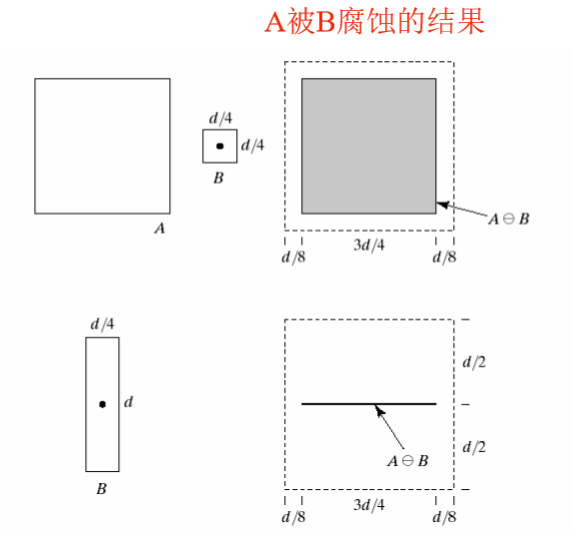A2 = imerode(A, se)

f = imread('D:\数字图像处理\第九章学习\Fig0908(a).tif');
se = strel('disk', 10);
g = imerode(f, se);
se = strel('disk', 5);
g1 = imerode(f, se);
g2 = imerode(f, strel('disk', 20));
subplot(2,2,1), imshow(f);title('(a)原始图像的尺寸为480x480像素:');
subplot(2,2,2), imshow(g);title('(b)用半径为10的圆形腐蚀:');
subplot(2,2,3), imshow(g1);title('(c)用半径为5的圆形腐蚀:');
subplot(2,2,4), imshow(g2);title('(d)用半径为20的圆形腐蚀');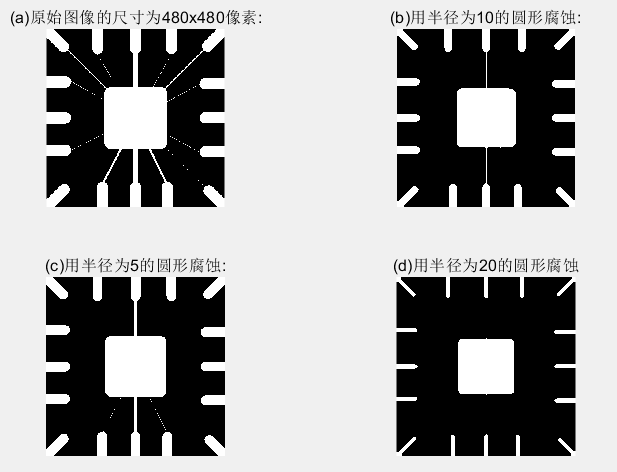## （三） 膨胀与腐蚀的结合

#### 3.1 开操作和闭操作

1. 使图像的轮廓变得光滑，断开狭窄的间断和消除细的突出物。
2. 使用结构元素B对集合A进行开操作，定义为：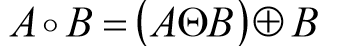先用B对A腐蚀，然后用B对结果膨胀。
3. 与开操作等价的数学表达式为: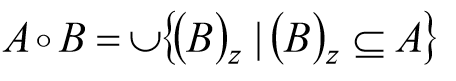4. A o B 的边界通过B中的点完成。
5. B在A的边界内转动时，B中的点所能到达的A的边界的最远点。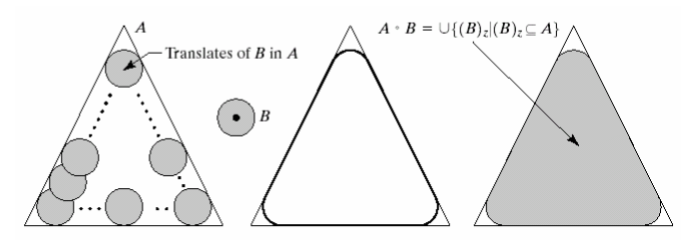6. A o B 是 A的子集合。
7. 如果C是D的子集，则 C o B是 D o B的子集。
8. (A o B) o B = A o B

1. 同样使图像的轮廓变得光滑，但与开操作相反，它能消除狭窄的间断和长细的鸿沟，消除小的孔洞，并填补轮廓线中的裂痕。
2. 使用结构元素B对集合A进行闭操作，定 义为：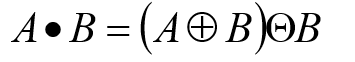先用B对A膨胀，然后用B对结果腐蚀。
3. A . B的边界通过B中的点完成 。
4. B在A的边界外部转动 :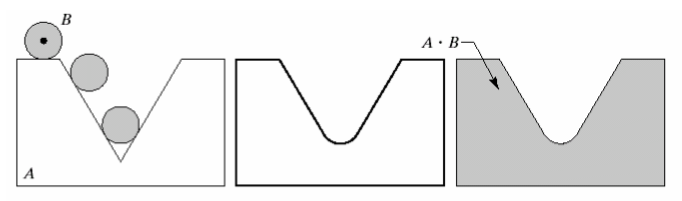5. A 是 A . B的子集合。
6. 如果C 是 D 的子集 ， 则C . B 是 D . B的子集。
7. (A . B) . B = A . B

C = imopen(A, B)

C = imclose(A, B)

A为二值图像，B为0，1矩阵组成，并且是指定结构元素。

f = imread('D:\数字图像处理\第九章学习\Fig0910(a).tif');
se = strel('square', 40);
fo = imopen(f, se);
fc = imclose(f, se);
foc = imclose(fo, se);
subplot(2,2,1), imshow(f), title('(a)原图');
subplot(2,2,2), imshow(fo), title('(b)开操作');
subplot(2,2,3), imshow(fc), title('(c)闭操作');
subplot(2,2,4), imshow(foc), title('(d) (b)的闭操作结果');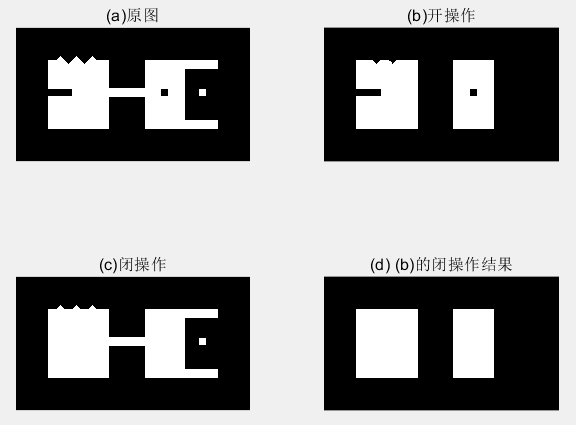1. 图(a)中的图像设计了一些用于演示开操作和闭操作的特征，比如细小突起、细的桥接点、几个弯口、孤立的小洞、 小的孤立物和齿状边缘。
2. 图 (b)显示了结果。注意，从图中可以看出，细的突出和外部点的边缘的不规则部分被去除掉了，细的桥接和小的孤立物也被去除了。
4. 图 (d)显示了平滑过的物体。

f = imread('D:\数字图像处理\第九章学习\Fig0911(a).tif');
se = strel('square', 6);
fo = imopen(f, se);
foc = imclose(fo, se);
subplot(1,3,1), imshow(f), title('(a)带噪声的指纹图像');
subplot(1,3,2), imshow(fo), title('(b)图像的开操作');
subplot(1,3,3), imshow(foc), title('(c)先用开操作，再用闭操作');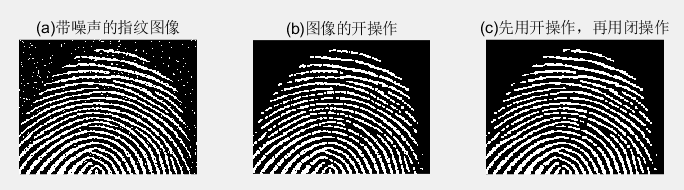1. 图(a)是受噪声污染的指纹二值图像，噪声为黑色背景上的亮元素和亮指纹部分的暗元素。
2. 图(b)所示的图像。发现，对图像进行开操作可以去除噪声点，但是这种处理在指纹的纹脊上又引入一些缺口
3. 图( c )显示了最终结果。在这个结果中，大多数噪声被消除了，开运算的闭运算可以给指纹填充缺口，但是指纹纹路并没有完全恢复 。

#### 3.2 击中或击不中变换

A中对B进行的匹配（击中）表示为：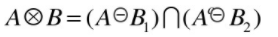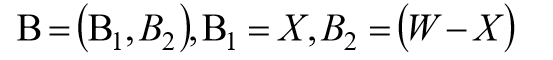B1是由与一个对象相联系的B元素构成的集合，B1是由与一个对象相联系的B元素构成的集合。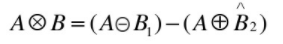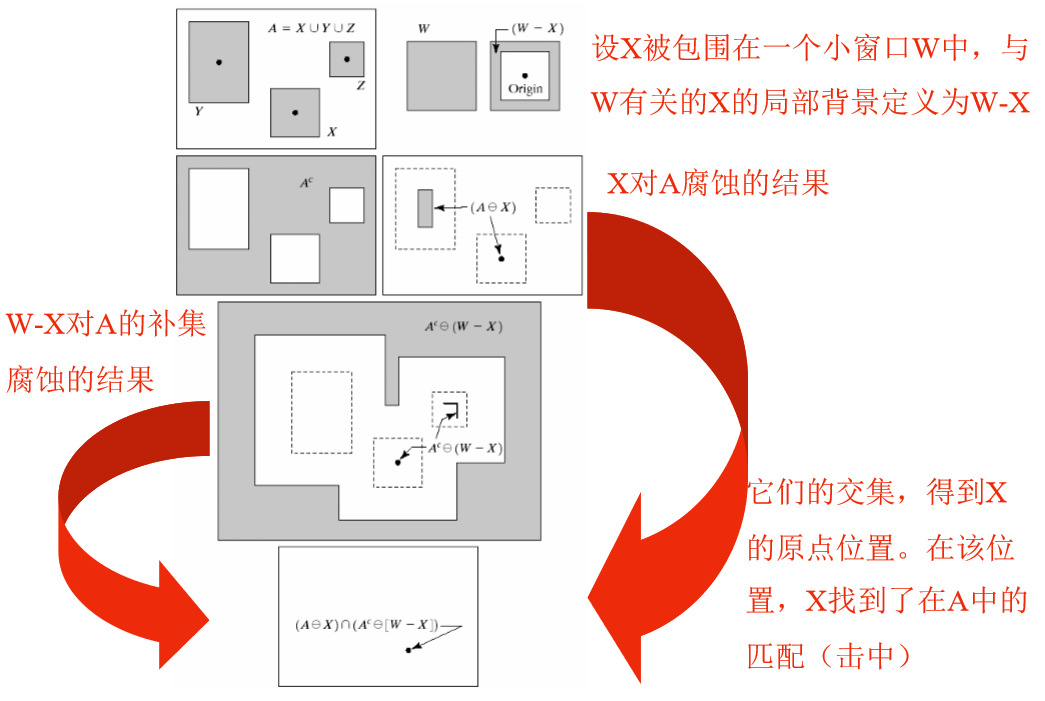C = bwhitmiss(A, B1, B2)

f = imread('D:\数字图像处理\第九章学习\Fig0913(a).tif');
B1 = strel([0 0 0;0 1 1; 0 1 0]);
B2 = strel([1 1 1;1 0 0;1 0 0]);
g = bwhitmiss(f,B1,B2);
subplot(1,2,1), imshow(f), title('(a)原始图像');
subplot(1,2,2), imshow(g), title('(b)击中、击不中变换的结果');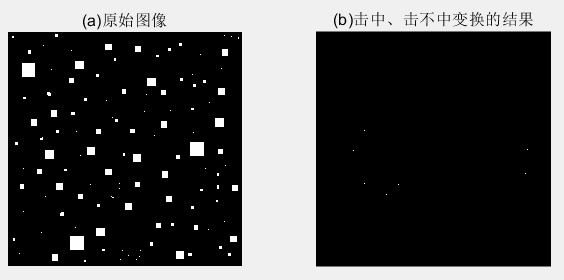1. 图(a)显示了包括各种尺寸的正方形图像。我们要定位有东、南相邻像素(这些 “击中”）和没有东北、北、西北、西和西南相邻像素(这些 “击不中”）的前景像素。这些要求导致以下B1,B2两个结构元。这两个结构元都不包括东南邻域像素，这称为不关心像素。用函数 bwhitmiss 来计算变换。
2. 图 (b)中的每个单像素点都是图 (a)中物体左上角的像素。图 (b)中是放大后的像素，以便更清晰。bwhitmiss的替代语法可以把Bl 和 B2 组合成间隔矩阵。只要 B1等于 1 或-1，B2 等于 1, 间隔矩阵就等于 1。对于不关心像素，间隔矩阵等于 0。

#### 3.3 bwmorph函数

g = bwmorph(f, operation, n);

f 是输入的二值图像，operation 是指定所希望运算的字符串，n 是指定重复次数的正整数。

f = imread('D:\数字图像处理\第九章学习\Fig0911(a).tif');
g1 = bwmorph(f, 'thin',1);
g2 = bwmorph(f, 'thin',2);
ginf = bwmorph(f,'thin', Inf);
subplot(1,4,1),imshow(f);title('(a)指纹图像:');
subplot(1,4,2),imshow(g1);title('(b)细化一次后的指纹图像:');
subplot(1,4,3),imshow(g2);title('(c)细化两次后的图像:');
subplot(1,4,4),imshow(ginf);title('(d)一直细化到稳定状态的图像：');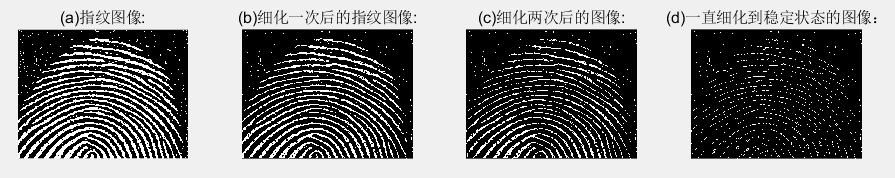f = imread('D:\数字图像处理\第九章学习\Fig0916(a).tif');
fs = bwmorph(f,'skel',Inf);
for k = 1:5
fa = fs & ~endpoints(fs);
end
subplot(1,3,1),imshow(f);title('(a)骨头图像:');
subplot(1,3,2),imshow(fs);title('(b)使用函数 bwmorph 得到的骨豁:');
subplot(1,3,3),imshow(fa);title('（c)使用函数 endpoint 裁剪后的骨豁：');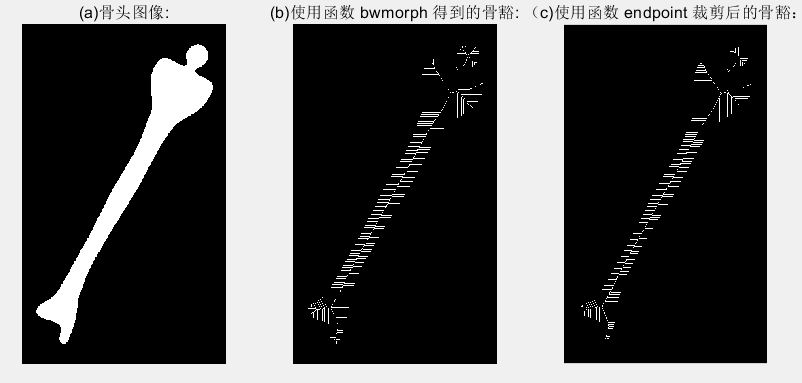## （四）标记连通分量

[L, num] = bwlabel (f, conn)

f 是输入二值图像，coon指定希望的连接方式（不是4连接就是8连接），输出L叫做标记矩阵，函数num则给出找到的连通分量总数。

f = imread('D:\数字图像处理\第九章学习\Fig0917(a).tif');
imshow(f);title('(a)标注连通分量原始图像:');
[L,n]=bwlabel(f);        %L为标记矩阵，n为找到连接分量的总数
[r,c]=find(L==3);        %返回第3个对象所有像素的行索引和列索引
rbar=mean(r);
cbar=mean(c);
figure,imshow(f);title('(b)标记所有对象质心后的图像:');
hold on            %保持当前图像使其不被刷新
for k=1:n
[r,c]=find(L==k);
rbar=mean(r);
cbar=mean(c);
plot(cbar,rbar,'Marker','o','MarkerEdgeColor','k',...
'MarkerFaceColor','k','MarkerSize',10);
plot(cbar,rbar,'Marker','*','MarkerFaceColor','w'); %其中的marker为标记
end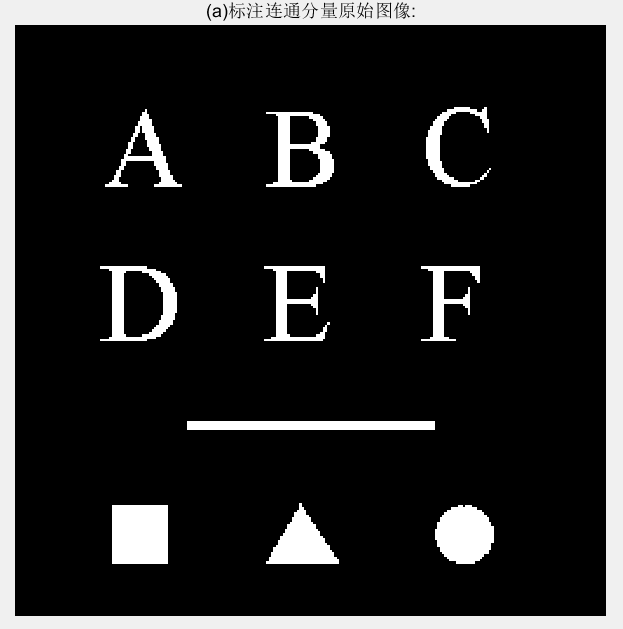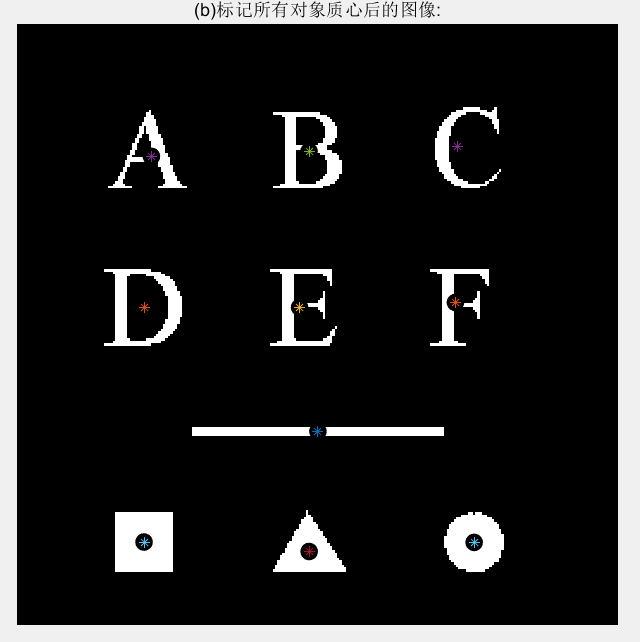## （五）形态学重建

1. 将h1初始化为标记图像f。
2. 创建结构元素 ：B = ones(3)。
3. 重复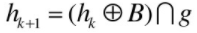直到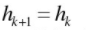其中，标记f必须是g的一个子集。

#### 5.1 通过重建进行开操作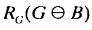f = imread('D:\数字图像处理\第九章学习\Fig0917(a).tif');
subplot(3,2,1),imshow(f);title('(a)重构原始图像');
fe=imerode(f,ones(51,1));%竖线腐蚀
subplot(3,2,2),imshow(fe);title('(b)使用竖线腐蚀后的结果');
fo=imopen(f,ones(51,1));%竖线做开运算
subplot(3,2,3),imshow(fo);title('(c)使用竖线做开运算结果');
fobr=imreconstruct(fe,f);%fe做标记
subplot(3,2,4),imshow(fobr);title('(d)使用竖线做重构开运算');
ff=imfill(f,'holes');%对f进行孔洞填充
subplot(3,2,5),imshow(ff);title('(e)对f填充孔洞后的图像');
fc=imclearborder(f,8);%清除边界，2维8邻接
subplot(3,2,6),imshow(fc);title('(f)对f清除边界后的图像');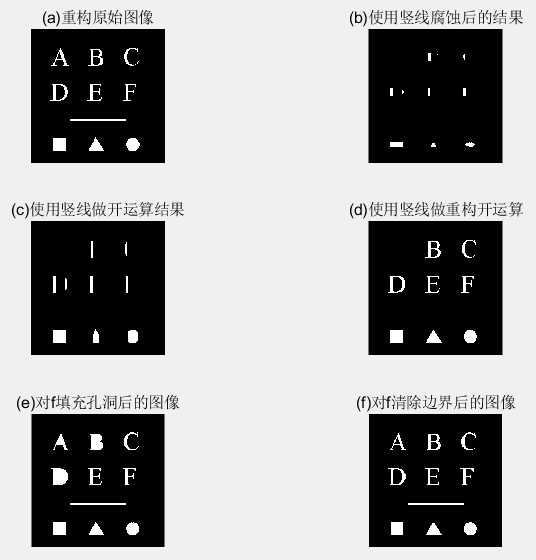1. 传统开运算中，腐蚀去除掉小对象，随后的膨胀恢复原始对象形状，但受元素结构影响，恢复的往往不是很精确。
2. 重构则能精确恢复原始图像。

#### 5.2 填充孔洞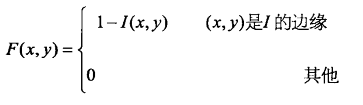g = imfill(f,‘holes’);

#### 5.3 清除边界物体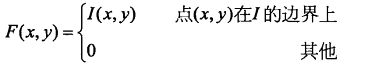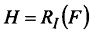g = imclearborder(f,conn)

f 是输入图像，g 是结果。conn 的值不是 4 就是 8(默认)。 物体更亮且与图像边界相连接的结构。

## （六）灰度级形态学

#### 6.1 膨胀和腐蚀

1. 使用结构元素b对f的灰度膨胀定义为：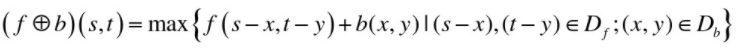其中，$D_f$$D_b$分别是f和b的定义域，f和b是函数而不是二值形态学情况中的集合。

2. 当结构元素b是平坦的，即b(x,y)在其定义域内都为0时：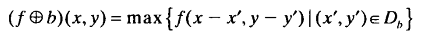1. 使用结构元素b对f的灰度腐蚀定义为：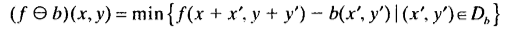其中，$D_f$$D_b$分别是f和b的定义域。

2. 当结构元素b是平坦的，即b(x,y)在其定义域内都为0时：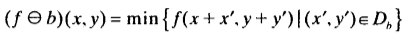f = imread('D:\数字图像处理\第九章学习\Fig0923(a).tif');
se=strel('square',3);  %构造了一个平坦的3x3的结构元素
gd=imdilate(f,se);    %对原图像进行膨胀操作
ge=imerode(f,se);     %对原图像进行腐蚀操作
subplot(3,2,1);imshow(f,[]);title('(a)原始图像');
subplot(3,2,2),imshow(gd,[]);title('(b)膨胀的图像');
subplot(3,2,3),imshow(ge,[]);title('(c)腐蚀的图像');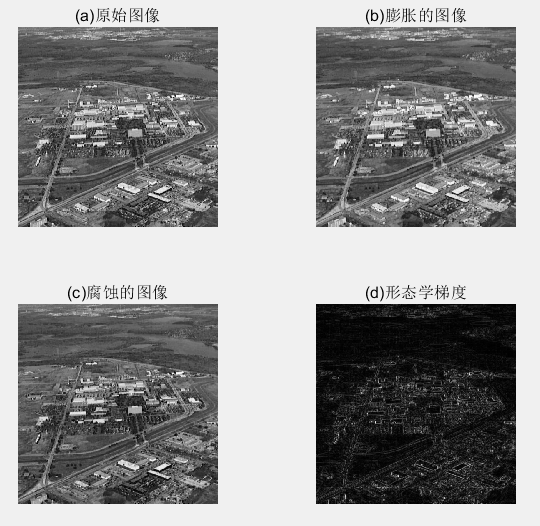1. 膨胀得到的图像比原图像更明亮，并且减弱或消除小的，暗的细节部分。即比原图像模糊。
2. 腐蚀得到的图像更暗，并且尺寸小，明亮的部分被削弱 。

#### 6.2 开操作和闭操作

1. 在灰度图像中，开操作的表达式与二值图像拥有相同的形式。2. 把一幅图像看做是一个三维表明，其亮度值代表xy平面上的高度值，则当结构元素b在f下面活动时，结构元素的任何部分的最高值构成了开运算的结果。
3. 先进行腐蚀操作可以除去小的亮的图像细节，但这样会使图像变暗，接下来进行膨胀操作增强图像的整体亮度。

1. 在灰度图像中，闭操作的表达式与二值图像拥有相同的形式。2. 当结构元素b在f的上面活动时，结构元素的任何部分的最低值构成了闭运算的结果 。
3. 先通过膨胀操作除去图像中的暗细节，同时增加图像的亮度，接下来对图像进行腐蚀，而不会将膨胀操作除去的部分重新引入图像中。

f = imread('D:\数字图像处理\第九章学习\Fig0925(a).tif');
subplot(3,2,1),imshow(f);
title('(a)木钉图像原图');
se=strel('disk',5);     %disk其实就是一个八边形
fo=imopen(f,se);        %经过开运算
subplot(3,2,2),imshow(f);
title('(b)使用半径5的disk开运算后的图像');
foc=imclose(fo,se);
subplot(3,2,3),imshow(foc);
title('(c)先开后闭的图像');
focd=imclose(f,se);
subplot(3,2,4),imshow(focd);
title('(d)原始图像的闭操作');
foce=imopen(focd,se);
subplot(3,2,5),imshow(foce);
title('(e)先闭后开的图像');
fasf=f;
for i=2:5
se=strel('disk',i);
fasf=imclose(imopen(fasf,se),se);
end
subplot(3,2,6),imshow(fasf);
title('(f)使用开闭交替滤波后图像');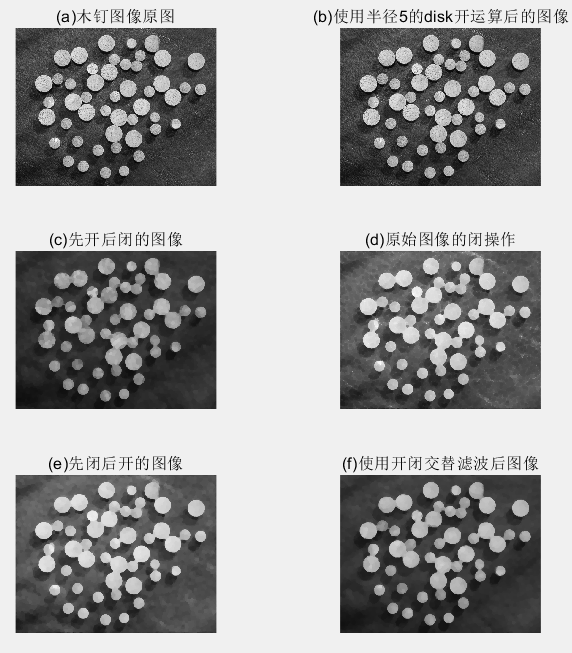1. 图 (b)显示了开操作的图像 fo, 在这里，我们看到，亮区域己经被调低了(平滑)，木钉上的暗条文几乎没有受影响。
2. 图 (c )显示了开操作的闭操作 foe。现在我们注意到，暗区域已经被平滑得很好了，结果是整个图像得到全部平滑。这种过程通常叫做开-闭滤波。先开运算后闭运算构成噪声滤波器，用来平滑图像并去除噪声。
3. 图 (d)显示了原始图像的闭操作结果。木钉上的暗条文已经被平滑掉了，主要留下了亮的细节(注意背景中的亮条文)。
4. 图 (e)显示了这些条文的平滑和木钉表面的进一步平滑效果。最终结果是原始图像得到全部平滑。

f = imread('D:\数字图像处理\第九章学习\Fig0926(a).tif');
g = f>=(255*graythresh(f));
se=strel('disk',100);
fo=imopen(f,se);
f2=imsubtract(f,fo);
g1 = f2>=(255*graythresh(f2));
subplot(2,3,1),imshow(f);
title('(a)原始图像');
subplot(2,3,2),imshow(g);
title('(b)经过阈值处理后的图像');
subplot(2,3,3),imshow(f);
title('(c)原图开运算后的图像');
subplot(2,3,4),imshow(f2);
title('(d)原图减去开运算');
subplot(2,3,5),imshow(g1);
title('(e)最终结果');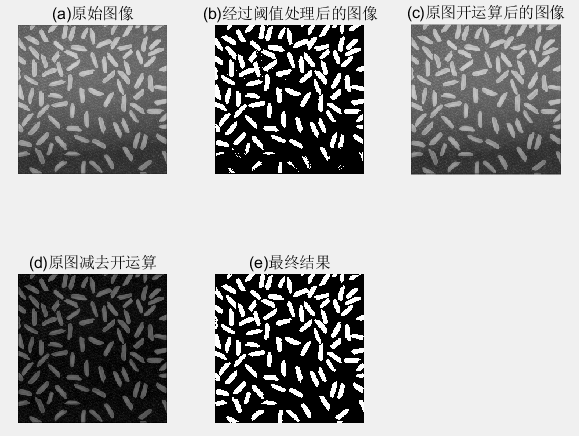1. 图 (a) :显示了一幅米粒的图像f，图像下部的背景比上部的黑。这样的话，对不平坦的亮度进行阈值处理会很困难。
2. 图 (b) "是阈值处理方案，图像顶端的米粒被很好地从背景中分离开来，但是图像底部的米粒没有从背景中正确地提取出来。
3. 图(c ):对图像进行开操作，可以产生对整个图像背景的合理估计。
4. 图(d) :把图(c )从原始图像中减去，生成一幅拥有合适的均勾背景的米粒图像.
5. 图(e):显示了新的经阈值处理后的图像。注意，改进效果超过了图 (b)。

f = imread('D:\数字图像处理\第九章学习\Fig0926(a).tif');
sumpixels=zeros(1,36);
for k=0:35
se=strel('disk',k);
fo=imopen(f,se);
sumpixels(k+1)=sum(fo(:));
end
%可以看到，连续开运算之间的表面积会减少
plot(0:35,sumpixels),xlabel('k'),ylabel('surface area');
title('(a)表面积和结构元素半径之间的关系');
figure,plot(-diff(sumpixels));%diff()函数为差分或者近似倒数，即相邻2个之间的差值
xlabel('k'),ylabel('surface area reduction');
title('(b)减少的表面积和结构元素半径之间的关系');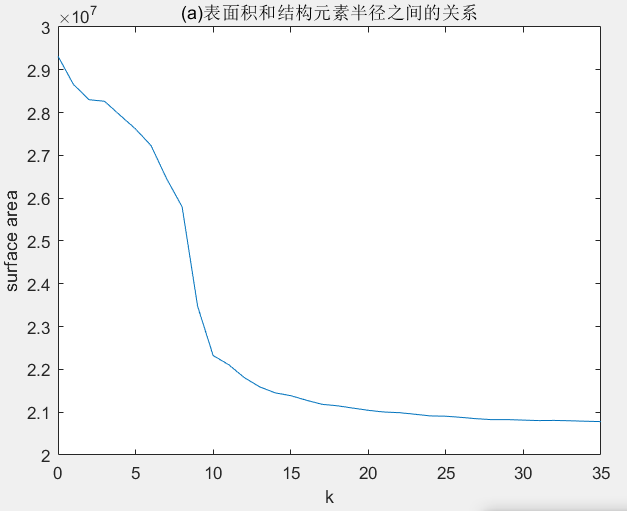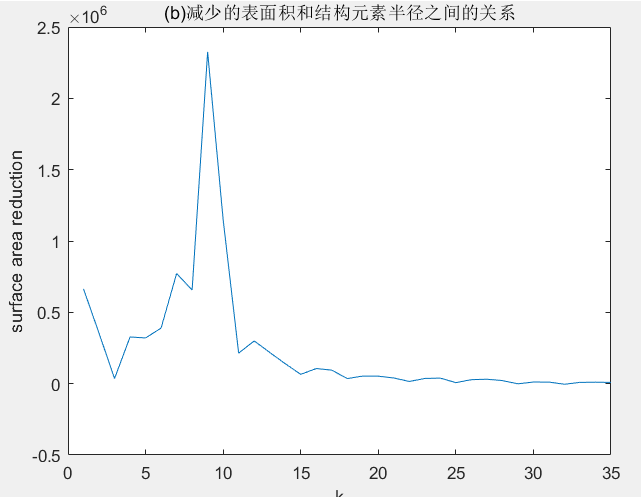1. (a)连续开运算之间的表面积会减小。
2. (b)图峰值表明出现了大量的有着这种半径的对象。

#### 6.3 重建

1. h极小值变换：标记图像是由掩膜挑选ing减去常量所得。
2. 开运算重建：先腐蚀后重建。
3. 闭运算重建：对图像求补、计算其开操作重建并对结果求补。

f = imread('D:\数字图像处理\第九章学习\Fig0930(a).tif');
subplot(3,3,1),imshow(f);
title('(a)原图像');
f_obr=imreconstruct(imerode(f,ones(1,71)),f);
subplot(3,3,2),imshow(f_obr);
title('(b)重建的开操作');
f_o=imopen(f,ones(1,71));
subplot(3,3,3),imshow(f_o);
title('(c)开操作');
f_thr=imsubtract(f,f_obr);    %顶帽重构
subplot(3,3,4),imshow(f_thr);
title('(d)重建的顶帽操作');
f_th=imsubtract(f,f_o)    %标准顶帽运算，方便比较
subplot(3,3,5),imshow(f_th);
title('(e)顶帽操作');
g_obr=imreconstruct(imerode(f_thr,ones(1,11)),f_thr);
subplot(3,3,6),imshow(g_obr);
title('(f)用水平线对(b)经开运算后重建图');
g_obrd=imdilate(g_obr,ones(1,2));
subplot(3,3,7),imshow(g_obrd);
title('(g)使用水平线对(f)进行膨胀');
f2=imreconstruct(min(g_obrd,f_thr),f_thr);
subplot(3,3,8),imshow(f2);
title('(h)最后的重建结果');